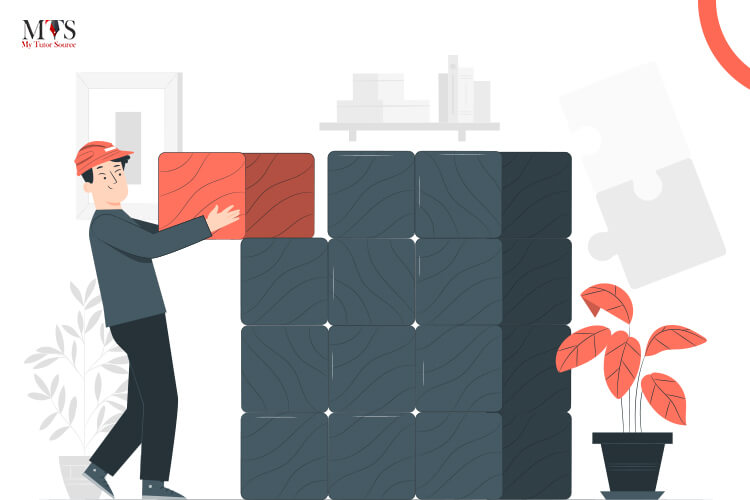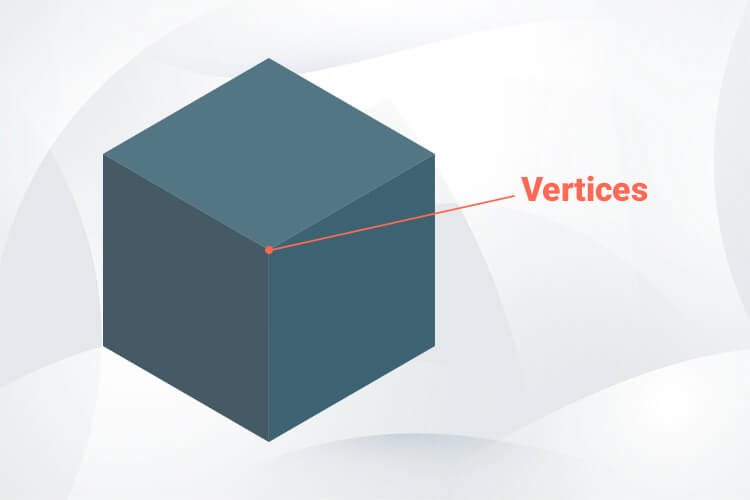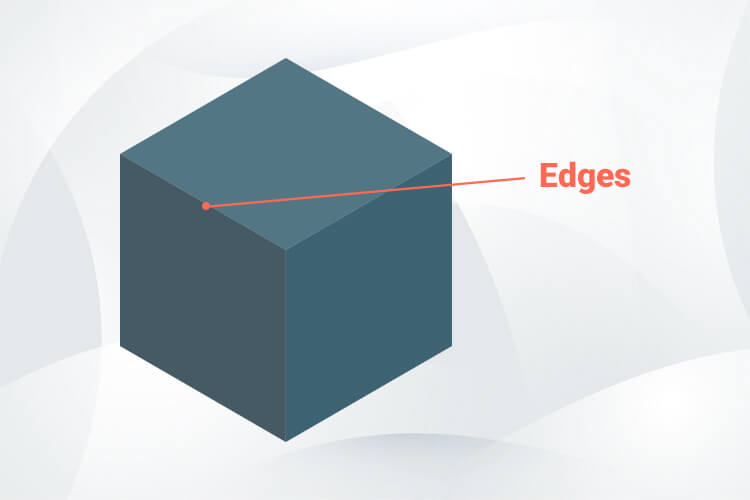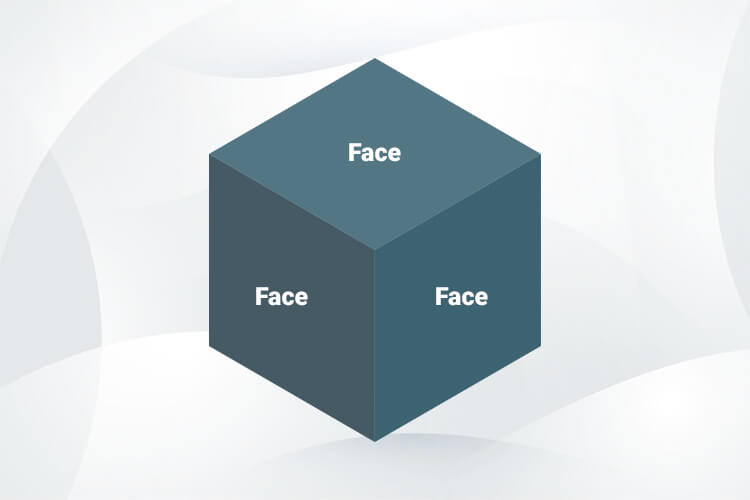# Vertices, Edges, and Faces: Explanations, Examples, and Euler’s FormulaMath doesn’t always have to be hard! It’s a subject that helps you even after school/college is over! The best part of this subject is that if you master the basics you can get hang of it and start scoring good grades in exams.

Vertices, edges, and faces are the basics of geometry. And, without getting to know the main differences between them, students might fail to solve the high-level equations.

To help students learn all about vertices, edges, and faces, we’ve devoted this blog to these parts of 2D/3D shapes. Below you’ll find easy explanations of these three parts of geometric shapes along with their examples.

Note: This blog can be used as a curriculum guide for primary-level students, as vertices, edges, and faces of 3D shapes are introduced to 2-year children.

## What Are Vertices?

Vertices, or in easy words “the corners” are the points where two line segments meet. The singular word for vertices is the vertex.## What are Edges?

Edges are the line segments that join two vertices. Almost all the 2d and 3d shapes have straight edges. But a few shapes like hemispheres, cones, etc., have curved edges.## What are Faces?

Faces are flat surfaces that are locked between the vertices and edges, as shown in the image below:## Examples of Vertices, Edges, and Faces

 Shape Names Vertices Faces Edges Cube (Square) 8 6 12 Pyramid 5 5 8 Cuboid (Rectangle) 8 6 12 Cone 1 2 1 Cylinder 0 3 2 Sphere 0 1 0

## Euler’s Formula

Euler’s formula is used to find the relationship between vertices, edges, and faces. This formula is written as:

F + V = 2 + E

Where F denotes faces, V denotes vertices and E denotes edges. Now, let’s have a look at the solved example in the next section.

## Solved Example

Q: Let’s say a cube has 6 faces, 12 edges and 8 vertices is a cube. Use Euler’s formula for the cube.

Solution:

Given, F= 6

E=12

V=8

Thus,, according to Euler’s formula,

F + V – E = 2

Taking L.H.S.,

6 + 8 – 12

= 14 – 12

= 2 (R.H.S)

Hence, proved.

## FAQs

### How many edges does a cone have?

A cone has one 1 curved edge.

### How many faces are there in a cuboid?

There are 6 faces in a cuboid.

### Find Top Tutors in Your AreaWith over 3 years of experience in teaching, Chloe is very deeply connected with the topics that talk about the educational and general aspects of a student's life. Her writing has been very helpful for students to gain a better understanding of their academics and personal well-being. I’m also open to any suggestions that you might have! Please reach out to me at chloedaniel402 [at] gmail.com